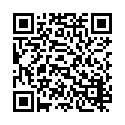Symbolab - Math solver Pro v9.3.1

Our math photo answer &amp; scanner solves any math problem with steps. Have a private math tutor in your pocket!

Symbolab has been used by over 200 million students to work through and understand more than one billion math problems and explanations.

Equations, integrals, derivatives, limits, and much more.

Symbolab Math Solver app is composed of hundreds of Symbolab's most powerful calculators:
Graphing Calculator
Fractions Calculator
Equation Calculator
Integral Calculator
Derivative Calculator
Limit calculator
Inequality Calculator
Trigonometry Calculator
Matrix Calculator
Functions Calculator
Series Calculator
ODE Calculator
Laplace Transform Calculator

Symbolab Math Solver solves any math problem including Pre- Algebra, Algebra, Pre-Calculus, Calculus, Trigonometry, Functions, Matrix, Vectors, Geometry and Statistics.

Symbolab is a global leader in education technology with over 200 million users worldwide. Symbolab is committed to helping students learn math, providing step-by-step math answers to any math problem, as well as AI-driven personalized learning, assessments, insights, and more.
User Reviews
Based on 0 Votes and 0 User Reviews
5 Star
0
4 Star
0
3 Star
0
2 Star
0
1 Star
0